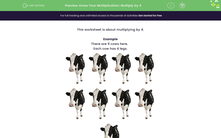# Multiply by 4, using repeated addition

In this worksheet, students learn to multiply by 4.Key stage:  KS 2

Curriculum topic:   Number: Multiplication and Division

Curriculum subtopic:   Use Multiplication/Division Facts (3, 4 and 8)

Difficulty level:#### Worksheet Overview

In this activity, we will learn about multiplying by 4.

To multiply by four, we can use repeated addition.

Here is a cow. One cow has 4 legs.Let's think about what happens if we have 5 cows, each with 4 legs and we want to find the total number of legs!We could count the legs one by one but this would take a while!

5 groups of 4 looks like this as repeated addition

4 + 4 + 4 + 4 + 4 = 20

There are 20 legs in total!

Now let's try an example of multiplying by 4, using repeated addition.

Example

Here are 4 cows. They each have 4 legs. How many legs in total?In total, there are 4 + 4 + 4 + 4 = 16 legs.

What is 4 times 4?

4 × 4 = 16

Now that you understand repeated addition, let's try some more questions.

### What is EdPlace?

We're your National Curriculum aligned online education content provider helping each child succeed in English, maths and science from year 1 to GCSE. With an EdPlace account you’ll be able to track and measure progress, helping each child achieve their best. We build confidence and attainment by personalising each child’s learning at a level that suits them.

Get started